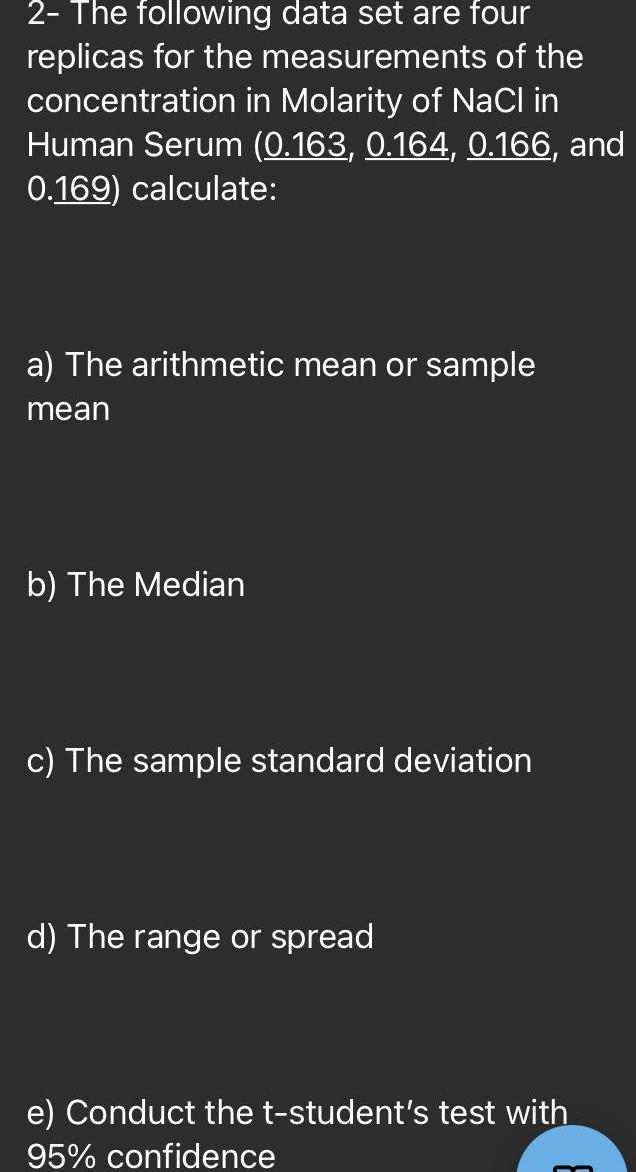Question:

# 2- The following data set are four replicas for the

Last updated: 7/10/20222- The following data set are four replicas for the measurements of the concentration in Molarity of NaCl in Human Serum (0.163, 0.164, 0.166, and 0.169) calculate: a) The arithmetic mean or sample mean b) The Median c) The sample standard deviation d) The range or spread e) Conduct the t-student's test with 95% confidence